ネコと趣味ときどき仕事

TOP >  天文 >  View inside a BlackHole

## View inside a BlackHole

A view inside a cosmic black hole and the mechanism that creates a closed space.

Physics Original Theory Research Series (My Hypothesis)  →Japanese edishon

←  returns to the top page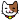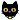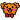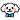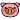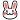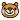We are observers

This paper will be a logical development assuming that you have some understanding of my theory of " the formation of the universe, the story of the birth of the universe ". The rough content of this is completely paradoxical cosmology with "big bang cosmology". → " Cosmic Black Hole Hypothesis "

It assumes that our cosmic space is a falling process within the Schwarzschild radius of a huge cosmic black hole in the outer space, which is an unobservable region for us.

1. Theories and assumptions used to construct this thesis

This thesis builds logic assuming that the following theories and premises are correct. I consider the following to be the standard theory, but it may contain some extensions of mine. But it shouldn't deviate too much.

1-1. Phenomenon when an observer approaches a massive star (between two objects)

Light travels straight in a vacuum space. The reason why light is observed bending in a gravitational field is because three-dimensional space is bending. Light travels straight.
Let A be a massive star and B be an observer. Suppose that the light emitted from B is bent by the gravitational field of A , and the optical path that was originally supposed to go to a place other than A is now connected to A. In this case, A exists equidistant on the linear extension of the optical path from B connected to A.

Since there are an infinite number of actual optical paths, when viewed from B, A appears to spread. Moreover, this is not only "visible", but it is actually a curved three-dimensional spatial structure in which A spreads out and wraps around B at equal distances. The gravitational action of A spreads out as well. But the absolute amount of gravity does not change. The gravitational potential of B is determined by the mass of A and the distance between A⇔B . Since the direction in which gravity is received is a vector sum, it is the direction in which the optical path in the figure is a straight line.

This reasoning applies when the light arrival time is so short that it can be ignored. For scales such as 10 billion years one way, see " 4. Considerations on optical paths".

1-2. how light travels

(2) In a curved three-dimensional space, there may be an infinite number of optical paths connecting two points A ⇔ B. However, the optical paths are all the shortest distances (straight lines) connecting the two points A⇔B, and are all equidistant. This is the same as that there are countless shortest routes connecting the north pole and the south pole on the surface of the earth, which is a curved two-dimensional surface, and all of them are equidistant.

1-3. Situation when falling within the Schwarzschild radius of a massive star

Suppose A is a supermassive cosmic nucleus and a black hole with a huge Schwarzschild radius of hundreds of billions of light-years or more. And let B be an observer in free fall within the Schwarzschild radius.

Within the Schwarzschild radius, light paths in all directions emanating from B cannot escape to the outside. Therefore, all optical paths are bent and lead to the central core of the universe.

That is, all optical paths in all directions emitted from the falling observer B are connected to the central nucleus A. Here, the optical paths between the two points A ⇔ B are all the shortest distances, and are equidistant. Therefore, when viewed from the observer B, the spatial structure is such that the central nucleus A exists equidistantly in all directions.

In other words, the observer B is wrapped in the surface of the central nucleus A and becomes a closed space completely separated from the external space. It is due to this spatial structure that we cannot escape from the black hole and cannot communicate with the outside world.

In addition, since the cosmic nucleus exists at an equal distance in all directions, its gravity is canceled and becomes weightless, and the observer B falling there becomes inertial motion (uniform motion) instead of accelerated motion.

This starts when the Schwarzschild radius is exceeded. In this case, the fall observer B's fall energy does not increase and it descends the gravitational gradient of the central nucleus A, and finally collides with the central nucleus A. It is not common knowledge that the fall energy does not increase even if it descends the gravitational gradient, but this interpretation avoids the contradiction that the falling object gains infinite fall energy, and the law of conservation of mass and energy is observed. Moreover, the problem that a singular point is generated by infinite compression can be avoided.

An event horizon is formed between the core A and the falling observer B. The event horizon seen from B is the place where the light energy emitted there reaches zero and reaches B. It is also the place where the redshift becomes infinite.

Since the distances between A and B are equal in all directions, the distances to the event horizon are also equal. In other words, when viewed from the observer B, the event horizon exists at equal distances in all directions. This would appear to observer B as if the entire sky was enveloped in a dark, absolute zero temperature event horizon, with B floating in the center. This is a true representation of our situation in the real universe.

2. Illustrated details of how a closed space inside a cosmic black hole is created

1-1.

When an observer observes a star, if the star's mass is not very large and the bending of the light path due to gravity is negligible, the star appears to be the same size. The same is true if the distance is far enough.

1-2.

As an observer approaches a massive star, the gravitational field bends the path of light (the path of light), causing the star to appear larger than it actually is due to gravitational lensing. As explained in the figure below, stars can be seen on the straight line extension of the light that reaches the observer, so if you draw a picture, you can see that it will be greatly enlarged.

※Note: The optical path curve in the figure is not accurate. But the accuracy of the curves does not affect the conclusions of this paper. What matters is where light emitted in a certain direction ends up.

1-3.

As the observer gets closer to the massive star, the light path is bent even more and spreads out to cover the observer. In the lower image, most of the field of view will be occupied by massive stars.

※Note: The optical path curve in the figure is not accurate. But the accuracy of the curves does not affect the conclusions of this paper. What matters is where light emitted in a certain direction ends up.

1-4 .

As the observer gets closer to the massive star, the light path is bent even more, and it wraps around the observer behind the observer.

※Note: The optical path curve in the figure is not accurate. But the accuracy of the curves does not affect the conclusions of this paper. What matters is where light emitted in a certain direction ends up.

1-5.

As the observer approaches a massive star and falls past its Schwarzschild radius, the surface of the massive star completely envelops the observer, creating a closed space with no way out.

1-6.

Massive stars are not actually visible in all directions. An event horizon is formed equidistant between the massive star and the observer, completely enveloping the observer, and the observer appears to be floating in the center.
If we were to observe it as if it were floating in the center of an event horizon that was pitch black and the temperature was absolute zero, it would be the same as in our universe.

3. View inside a cosmic black hole

The inside-out space inverted by the cosmic black hole's enormous gravity, that is, the black hole's central nucleus covers the entire sky, and the event horizon in front of it is wrapped in a pitch-black, closed space near absolute zero that we can see. It is the universe recognized by

The distance to near the event horizon is estimated to be about 13.8 billion light years.

Our galaxy is also falling at a very high speed, so the distance to the central nucleus is shrinking at a constant speed. Along with this, the distance to near the event horizon, which is farthest from us, is also shortening.

When falling from infinity without obstacles, the falling speed reaches the speed of light at the Schwarzschild radius, but in fact most of the kinetic energy in the falling process becomes heat energy and is used to keep the external space at a high temperature. Therefore, the falling speed of our universe is also high, but it is considerably slower than the speed of light.

Other galaxies are also falling at about the same speed as us, so the relative distance does not change.

In the above figure, the central core of the universe is illustrated for convenience, but our outer space ends at the event horizon, and the central core beyond it does not exist in our world.

4. Light path considerations

The light path is reversible. If the light emitted from B is bent to create an optical path to reach A, conversely, the light emitted from A can go to B through the same optical path. However, while this is fine on normal time scales, on cosmic scales it is common to take 10 billion years or more one way.

Light from our current spatial coordinates travels to the central nucleus through the optical path reserved by the spatial fabric. But it will be several tens of billions of years before it arrives.

Light from a distant galaxy reaches us today by following this reserved path in reverse. The light that was emitted thousands, hundreds of millions, billions of years ago finally reaches us.

In other words, the reserved optical path that connects us to the core exists only at that moment, and the light emitted by the celestial bodies that existed in the past on that optical path reaches us over a long period of time. We are looking at the scenery of the past. But this is the fixed present tense, the real world.

Specifically, the optical path from the light emitted from our galaxy to the central nucleus is the basis. This is the future tense and is called a reserved optical path.

We are observing the light that has flowed back through this reserved optical path. But from the core to the event horizon is not our world. What we can barely observe is the light coming from celestial bodies on our reserved light path near the event horizon.

スポンサーサイト